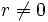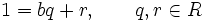# Element of minimum norm in Euclidean ring is a unit

## Statement

Suppose$R$ is a Euclidean ring, i.e.,$R$ is a commutative unital ring with a Euclidean norm$N$. Suppose$b \in R$ is a nonzero element such that:$N(b) \le N(r) \ \forall \ r \ne 0$.

Then,$b$ is a unit in$R$.

In particular, all elements of norm zero are units.

## Proof

Given: A commutative unital ring$R$ with Euclidean norm$N$, such that$N(b) \le N(r)$ for all$r \ne 0$.

To prove:$b$ is a unit in$R$.

Proof: By the Euclidean algorithm, we can write:$1 = bq + r, \qquad q,r \in R$

where$r = 0$ or$N(r) < N(b)$. By the assumption,$N(r) < N(b)$ is impossible, so we are forced to have$r = 0$. Thus:$1 = bq$,

and we obtain that$b$ is a unit.